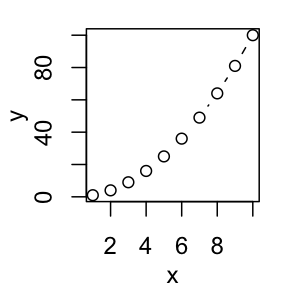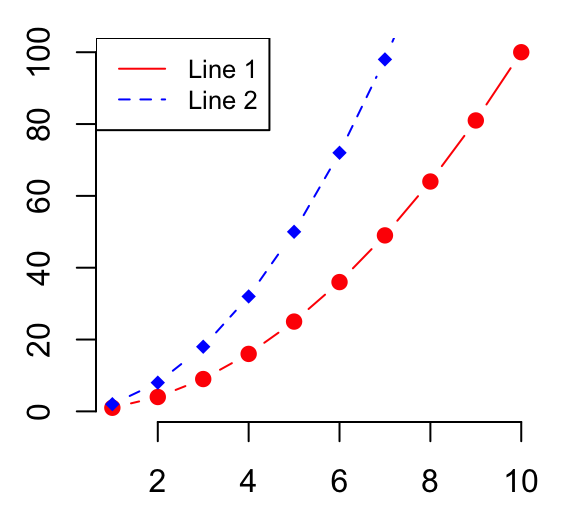# R Base Graphs

Previously, we described the essentials of R programming and provided quick start guides for importing data into R.

This chapter contains articles describring how to visualize data using R base graphs.

# Creating and saving graphs

• Creating graphs
• Saving graphs
• File formats for exporting plotscreate and save plots

Read more: —> Creating and Saving Graphs in R.

# Generic plot types in RRead more: —> Generic plot types in R.

# Scatter plots

• R base scatter plot: plot()
• Enhanced scatter plots: car::scatterplot()
• 3D scatter plots# Scatter plot matrices

• R base scatter plot matrices: pairs()
• Use the R package psychRead more —> Scatter Plot Matrices.

# Box plots

• R base box plots: boxplot()
• Box plot with the number of observations: gplots::boxplot2()# Strip Charts: 1-D scatter PlotsRead more —> Strip Charts: 1-D scatter Plots.

# Bar plots

• Basic bar plots
• Change group names
• Change color
• Change main title and axis labels
• Stacked bar plots
• Grouped bar plots# Line plots

• R base functions: plot() and lines()
• Basic line plots
• Plots with multiple lines# Pie charts

• Create basic pie charts: pie()
• Create 3D pie charts: plotix::pie3D()# Histogram and density plots

• Create histogram plots: hist()
• Create density plots: density()
``##  48.96467 56.38715 60.42221 43.27151 57.14562 57.53028``Read more —> Histogram and Density Plots.

# QQ-plots: Quantile-Quantile plots

Use qqPlot() [in car package]:Read more —> QQ-plots: Quantile-Quantile plots.

# Dot charts

• R base function: dotchart()
• Dot chart of one numeric vector
• Dot chart of a matrix# Plot group means and confidence intervalsRead more —> Plot Group Means and Confidence Intervals.

# R base graphical parameters

• Add an axis to a plot
• Change axis scale : minimum, maximum and log scale
• Customize tick mark labels
• Change plotting symbols
• Change line types
• Change colors

Read more —> R base graphical Parameters.

# Infos

This analysis has been performed using R statistical software (ver. 3.2.4).